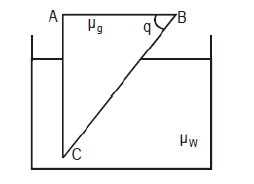# CBSE Class 12 Physics Chapter 9 - Ray Optics Important Questions

## Subjective Questions

Question 1.
Write the basic assumptions used in the derivation of lens – maker’s formula and hence derive this expression.

Question 2.
Derive a relation between focal length of a double convex lens and its radii of curvature.

Question 3.
Define the dispersive power of an optimal medium.Obtain a formula for the dispersive power of the material of a prism in terms of refractive index.

Question 4.
Derive the relation between the distance of object,distance of image and radius of curvature of convex spherical surface,when refraction takes place from rarer to denser medium and image formed is real. State the assumption and convention of sign used

Question 5.
What is achromatic lens? Deduce the condition for achromatic combination of two lenses

## Numerical Questions

Question 1.
An object is placed in front of convex lens made of glass. How does the image distance vary if the refractive index of the medium is increased in such a way that still it remains less than the glass?

Question 2.
Draw a labelled ray diagram showing the formation of image using a Newtonian type reflecting telescope.

Question 3.
What is the value of refractive index of medium of polarizing angle 60 degree?

Question 4.
A compound microscope consists of an objective of focal length 1cm and eye piece of focal length 5cm separated by 12.2cm. (a) At what distance from the objective should an object be placed so that the final image is formed at least distance of distinct vision? (b) Calculate the angular magnification in this case.

Question 5.
Calculate the value of θ, for which light incident normally on face AB grazes along the face BC. $\mu _g = 3/2$, $\mu _w = 4/3$.Question 6.
The focal length of a convex lens in air is 20 cm, what will be its focal length if it is immersed in a liquid of refractive index 1.35? Refractive index of glass is 1.50

Question 7.
Two 28 cm focal length converging lens are placed 16.5 cm apart.An object is placed 36 cm in front of one lens.Where will be the final image formed by the second lens be located. What is the total magnification
real, 22.3 cm beyond second lens
-0.713 inverted

Question 8.
Draw a ray diagram to show the formation of the real image of a point object due to a convex spherical refracting surface, when a ray of light is travelling from a rarer medium of refractive index $\mu _1$ to a denser medium of refractive index $\mu _2$. Hence derive the relation between object distance, image distance and radius of curvature of the spherical surface.

Question 9.
A concave mirror of radius of curvature 1m is placed at bottom of a tank of water. The mirror forms an image of the sun , when it is directly overhead. Calculate the distance of the images from the mirror for different depths, 80 cm and 40 cm of the water in the tank.
(Answer 50 cm , 47.5 cm)

Question 10.
A pin is placed 10 cm in front of convex lens of focal length 20 cm made of material of refractive index 1.5. The surface of lens farther away from the pin is silvered and has a radius of curvature 22 cm. Determine the position of the final image. Is the image real or virtual.

Question 11.
An object is placed 50 cm from the surface of a glass sphere of radius 12 cm along the diameter . Where will the final image be formed by reflection on both the surfaces? Refractive index of glass is 1.60

Question 12.
A bi-convex lens of radii of curvature 30.0 cm each is placed on the surface of water so that the lower surface is only immersed . An illuminated object 40 cm deep is observed vertically through the lens and water. Find the position of the image. Refractive index of air-glass=3/2 and of glass-air=4/3 .

Question 13.
An ant sitting 40 cm from a converging lens of focal length 20 cm moves to 1.00 m away from the lens. As it makes this move the distance of the image of the ant from the lens changes.
(a) Find the initial distance of image?
(b) Find the final distance of image?
(c) Is the ant images nearer to lens in final position?

Question 14.
A point object is moving along the principle axis of a concave mirror at rest of focal lenght 30 cm with a speed of 5 m/s. Find the speed of the image of object when the object is at the distance of 60 cm from the mirror